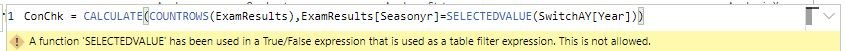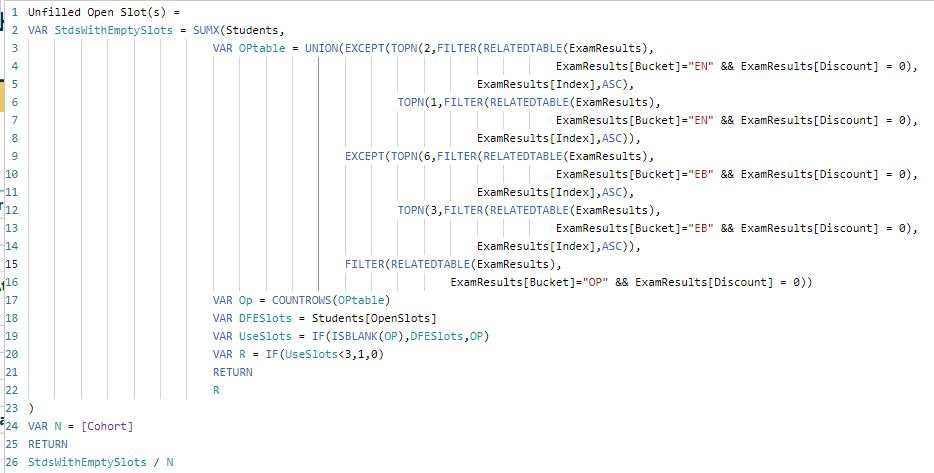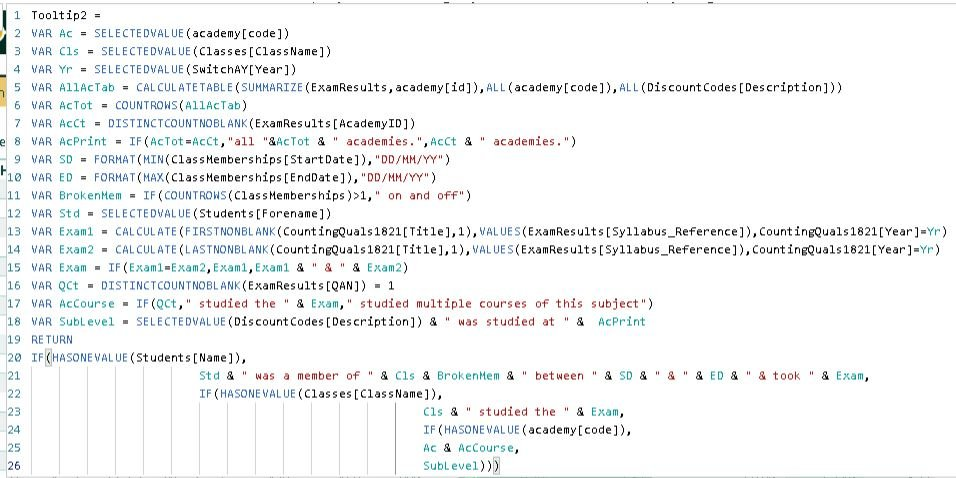# Use Variables to improve your DAX code

One of the hallmarks of getting better at BI is writing more complex DAX in columns and measures so I want to share with you guys by far the most helpful thing I´ve learned DAXwise - variables.

The concept is really simple - you give a name to a value or a table with some DAX and you can then refer to it by its name in a final calculation or another variable. We´ll do the syntax then I´ll give 5 reasons why this simple concept is actually awesome.

### Syntax

VAR name = expression
...repeat for as many variables as you like. Use Shift+Return to add new lines
RETURN
expression

e.g.

```    ```
Four =
VAR a = 2
VAR b = 2
RETURN
a+b
```
```

### 1. Troubleshooting

We all know the feeling when you write a big string of code, hit return and then you get nothing, or every kid has the same number and you know you´ve messed up. Using variables allows you to check the various elements of the calculation without touching the other steps.

For example, this is the formula for a measure from my last post on scatters:

```    ```
AllSubjects =
VAR SID = SELECTEDVALUE(Students[StudentId])
VAR SUB = SELECTEDVALUE(MergeValues[Subject])
VAR R = CALCULATE(
MAX(Merge1[Points]),
Merge1[StudentId]=SID,
Merge1[Subject]=SUB)
RETURN
R
```
```

The formula returns the variable R but that can be easily changed to check the values of SID or SUB (which are used to calculate R)

### 2. Readability

Naming the constituent parts of complex calculations is really useful in and of itself, especially, as often happens in education, they´re ones you do annually then have to refamiliarise yourself with.

Below is my formula for working out Progress 8 which has to gather a value for A8, then the estimated A8 value, subtract one from the other, divide by 10 - then (new in 2018!) check if the result is above a different minimum value. You can do this without variables but it´s much easier to follow when broken down into named steps.

```    ```P8 = AVERAGEX(
FILTER(Students,Students[IncludedP8]),
VAR Yr = SELECTEDVALUE(SwitchAY[Year])
VAR A8 = [A8_Total]
VAR Est = LOOKUPVALUE(P8Thresholds[A8],
P8Thresholds[Year],Yr,
P8Thresholds[KS2],Students[KS2FL])
VAR MinP8 = LOOKUPVALUE(P8Thresholds[MinP8],
8Thresholds[Year],Yr,
P8Thresholds[KS2],Students[KS2FL])
VAR Gross8 = A8 - Est
VAR P8 = Gross8 / 10
VAR AdjustedP8 = IF(P8 < MinP8,MinP8,P8)
RETURN
AdjustedP8)
```
```

More details on how to calculate Attainment 8

Note too that these variables are declared inside the AVERAGEX expression and are therefore calculated for each iteration through the students table.

### 3. Editing

Variables make copying and editing measures much easier too. Say you have a measure for testing if a kid passed English and Maths at Grade 4 – buried in that code somewhere are going to be two instances of "…>=4". When you copy the measure to make the Grade 5 and 7 versions you need to find and edit both conditions (and it won’t be obvious if you do one and forget the other). What I do is make the first line of code

VAR Threshold = 4

Then use >= Threshold as the comparator to my grade points. That way copying and editing the measure for different thresholds is much easier.

### 4. Context switching

Variables can also let you filter calculations if for whatever reason that filtering can not be provided via relationships.

The formula below returns an error, even though SwitchAY[Year] is a column with academic year values in it and it is in a slicer set to single select so will always contain a single value only. You'll get a similar error if you use a measure as a filter value in CALCULATEYou can make this formula work by first turning the SELECTEDVALUE expression into a value then using that as the filter

```    ```
ConChk =
VAR yr = SELECTEDVALUE(SwitchAY[Year])
RETURN
CALCULATE(COUNTROWS(ExamResults),ExamResults[Seasonyr]=yr)
```
```

### 5. Performance

The final, and possibly most important, reason to use variables is performance – they can significantly speed up your DAX queries.

Let’s take something relatively simple like the DfE’s classification of P8 scores, which is defined as Well Above Average if the lower confidence interval is above zero and the P8 value above 0.5, Above Average if the lci is above zero and so on through Average, Below Average and Well Below Average

Say you have measures [P8], [P8 Lower] and [P8 Upper] you can use the formulation SWITCH(TRUE(),logical test1,result 1,…alternate result) to classify them

(SWITCH(TRUE()… takes as its arguments boolean tests and result pairs and returns the result of the first test that evaluates to true. Performance wise it’s no different from using nested IFs it’s just easier semantically)

Let’s say you go for:

```    ```
P8Class =
SWITCH(TRUE(),
AND([P8 Lower]>=0,[P8]>=0.5), "Well Above Average",
[P8 Lower] > 0, "Above Average",
[P8 Upper] >= 0, "Average",
[P8]>= -0.5, "Below Average",
"Well Below Average")
```
```

From a performance point of view this is terrible because every time it gets to a condition with P8 in it it’s calculating P8 from scratch by going through every kid and all their results etc. The values are always the same in your current filter context so declaring them as

```    ```
VAR P8 = [P8]
VAR P8L = [P8 Lower]
VAR P8U = [P8 Upper]

RETURN
…
```
```

Is better but still not best – because the [P8 Lower] and [P8 Upper] measures still independently have to work out P8.

The optimum formula is

```    ```
VAR P8 = [P8]
VAR CI = [Confidence Interval]
VAR P8L = P8 – CI
VAR P8U = P8 + CI
```
```

It’s slightly more work for you (4 variables not 3), but it’s a lot less for the DAX engine as the last three are just arithmetic and the table iterations of students and assessment results that take real work is only happening once.

### Other cool things about variables

These didn't really fit the 5 reasons schema but are handy to know:

Variables can be tables as well as single (scalar) value. You don't need to do any different syntax, any expression that returns a table can be used as a table variable. For example this measure counts students who didn't fill all three Open slots for Attainment 8

The table variable OPtable lists (for each student) the exam results that can count in the Open bucket by combining the 2nd highest English result, the 4th, 5th and 6th EBacc results plus any that can only count as Open.

For students counting in my figures but who took their exams at other schools a different value populated from the DfE checking data (Students[OpenSlots]) is used.

Note that you can RETURN results multiple times. Here RETURN is used inside the SUMX iterator and to deliver the final result.Variables go especially well with logic and results depending on different contexts. I have a tool tip measure that works with a table that has subject, class and individual pupil results. At the subject level it tells me how many academies in the MAT did exams in the subject (adding all before the number if all of them), at the academy and class level it tells you which qualification(s) were taken and at the pupil level it tells you the dates the pupil was a member of the class and their qualification.

Here's the code (I use DiscountCodes[Description] to provide subject names as those used in schools are not necessarily standard across the MAT.